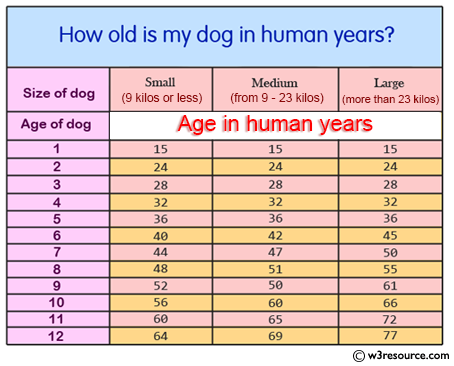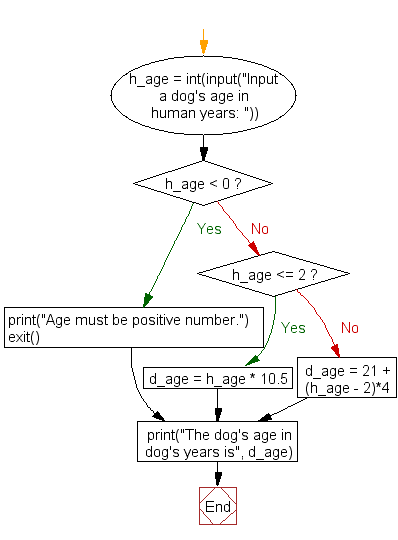﻿ Python Exercise: Calculate a dog's age in dog's years - w3resource# Python Exercise: Calculate a dog's age in dog's years

## Python Conditional: Exercise - 31 with Solution

Write a Python program to calculate a dog's age in dog's years.
Note: For the first two years, a dog year is equal to 10.5 human years. After that, each dog year equals 4 human years.

Pictorial Presentation:Sample Solution:

Python Code:

``````h_age = int(input("Input a dog's age in human years: "))

if h_age < 0:
print("Age must be positive number.")
exit()
elif h_age <= 2:
d_age = h_age * 10.5
else:
d_age = 21 + (h_age - 2)*4

print("The dog's age in dog's years is", d_age)
```
```

Sample Output:

```Input a dog's age in human years: 12
The dog's age in dog's years is 61
```

Flowchart :## Visualize Python code execution:

The following tool visualize what the computer is doing step-by-step as it executes the said program:

Python Code Editor :

Have another way to solve this solution? Contribute your code (and comments) through Disqus.

What is the difficulty level of this exercise?

Test your Python skills with w3resource's quiz

﻿

## Python: Tips of the Day

Returns a list with n elements removed from the beginning

Example:

```def tips_take(itr, n = 1):
return itr[:n]
print(tips_take([1, 2, 3], 5))
print(tips_take([1, 2, 3], 0))
```

Output:

```[1, 2, 3]
[]
```